The Number Cruncher
for SQL Server!

SQL Statistics Info:

"Total SQL Statistics can help your VB application deliver data quickly from your database to your consumers without having to re-enter or transform it."

- John Pearson, Visual Studio Magazine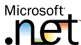NET Ready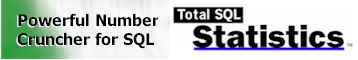# Total SQL Statistics Product Guide

## Product Highlights

### Powerful Statistical Analysis of Microsoft SQL Server Data

Go beyond the basic analysis of SQL queries. Total SQL Statistics offers a wide range of statistical functions to analyze your Microsoft SQL Server data. Data in tables, views, stored procedures, and user defined functions can be analyzed with the results in tables giving you maximum flexibility in working with these data sets.

Analysis scenarios are designed by running our interactive Scenario Designer program. Easily select the data set, fields, and analysis to perform. The settings are automatically saved. Run the analysis interactively to verify it's what you want. Then add it to your Visual Basic and Visual Studio .Net applications with a simple call into a distributable run-time library. Generate powerful statistical analysis without your users even knowing Total SQL Statistics is running in the background.

### Scenario Designer

The Total SQL Statistics Scenario Designer is where you create analysis scenarios. Scenarios contain information on the data to analyze and the statistical options to perform. A Wizard-like interface guides you through the process with no programming required. This allows you to perform powerful analysis with point-and-click ease. Your selections are automatically saved as "scenarios" which can be run interactively or invoked programmatically.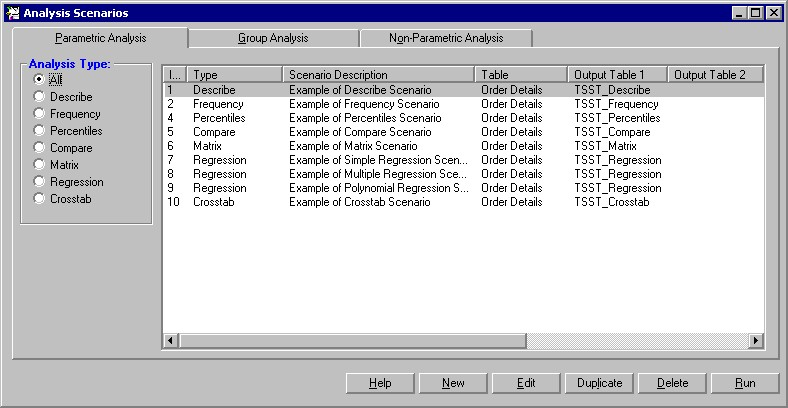Total SQL Statistics adds three small tables in your SQL Server database to store these scenarios.

### Programmatic Interface

Total SQL Statistics includes a programmatic interface for programmers who want to incorporate statistical functions directly into their applications. A DLL is all that is needed to add Total SQL Statistics to your applications—simply reference our DLL with the scenario you designed in the Scenario Designer, and the results are generated. You can specify all login information and direct output tables to a different database. While the analysis is running, events are available to let you know its progress. The analysis runs in the background, so your users won’t even know how you generate the complex analysis!

### Total SQL Statistics is .Net Ready!Total SQL Statistics supports Microsoft’s .Net platform. You can create Visual Studio .Net projects and include a reference to the Total SQL Statistics Runtime DLL just as you would in Visual Basic 6.

The Total SQL Statistics Code Generator makes it easy to insert the Visual Basic, VB .Net or C# code to run any scenario you define.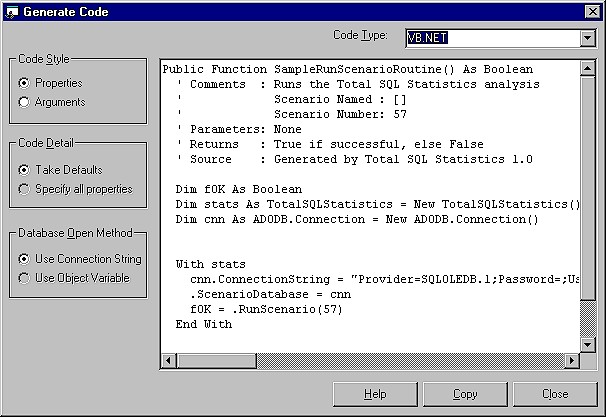## General Features

Total SQL Statistics includes a complete set of features to make it easy to add powerful data analysis to SQL Server projects.

 Feature Benefit Interactive Scenario Designer Create, test, and fine-tune statistical analysis interactively using a Wizard-like application. All scenarios are automatically saved for re-use or modification. Programmatic interface Easily add statistical analysis of SQL Server data to your Visual Basic and VB .Net projects. A hidden interface lets you run Total SQL Statistics while controlling what the user sees. Accuracy All calculations are in double precision (15 digits accuracy) Platform Support Runs on any network or operating system that supports SQL Server. Multi-user ready Multiple users can run an application that uses Total SQL Statistics at the same time. Royalty-Free License Each license allows a developer to include Total SQL Statistics in applications distributed to an unlimited number of customers. Small DLL Only one small file, a statistics ActiveX DLL under 1 MB in size, is added to your applications.

### Data Analysis Features

 Feature Benefit Analyze SQL Server Data Total SQL Statistics offers functions to analyze data stored in SQL Server tables, views, stored procedures, and user defined functions that return data. Results in SQL Server tables Rather than a large number of statistical functions, each returning one value, Total SQL Statistics generates many values at once. Each value is stored in a separate record and field in a table, making it easy for you to view, sort, query, or display the results. Analyze Large Data Sets Multiple fields and an unlimited number of records can be analyzed at one time. Group Data (optional) For every unique combination of values in the specified group fields, a separate calculation is generated. For instance, grouping on a State field generates separate results for each state (stored as individual records in the output table). Ignore Values (optional) Specify specific values or ranges of values to omit. For instance, 999 is sometimes entered as an "unknown" value and must be ignored. Weighting Field (optional) Specify a weighting field to perform calculations such as weighted averages, weighted standard deviations, weighted regressions, etc.

## Statistical Functions

The statistical functions are grouped into three categories: Parametric, Group, and Non-Parametric.

### Parametric Analysis Options

Parametric analysis is performed on numeric fields that are assumed to be continuous and normally distributed. Fields are analyzed individually or compared with each other.

 Type Description Describe Analysis of a numeric field: std. deviation, std. error, variance, coefficient of variance, skewness, kurtosis, geometric mean, harmonic mean, RMS, mode, confidence intervals, t-Test vs. mean, percentiles, etc. Frequency For each field, frequency distribution analysis for each interval (range of values): count, sum, percent of total, cumulative count, percent, and sum. Percentiles Median, quartiles, quintiles, deciles, and percentiles. Similar to Describe, but results placed in records rather than fields (each percentile is a record). Compare Compare two fields: mean and standard deviation of difference, correlation, covariance, R-square, paired t-Test. Matrix Similar to Compare, but rather than several fields compared to one, all fields are compared to each other creating a matrix. Regression Simple, multiple, and polynomial regressions with coefficient analysis, ANOVA, and residual table. Crosstab Cross-tabulation with row and column summaries, and % of row, column, and total for each cell. Chi-Square analysis is also available with expected value and % of expected for each cell.

### Group Analysis Options

Group analysis is the comparison of continuous, normally distributed numeric data between groups of records. A comparison field in the table defines the groups. For instance, you may want to compare data between men and women, or by race. Groups are usually of different sizes (number of records) unlike the Compare feature in Describe, which is for paired values.

 Type Description Two Sample t-Test Two Sample t-Test compares means between two groups of records. Calculations include pooled and separate t-values for the two groups. ANOVA Analysis of variance compares the means of multiple groups of records. Calculations include degrees of freedom, sum of squares within and between groups, F-value, and probability. Two way ANOVA Two-way analysis of variance compares multiple fields between groups of records. Same results as ANOVA with additional values for each additional field. Used to measure relative impact of each variable on the mean.

### Non-Parametric Options

Less powerful than parametric analysis, non-parametric analysis is used when the underlying data is not continuous, for instance ordinal data, or not normally distributed. Non-parametric analysis makes no assumption on the distribution of the underlying data, since the results are based on the ranks of the data. Non-parametric analysis can be made for each numeric field individually, compared with each other, or between groups of records (samples).

 Type Description Chi-Square One sample Chi-Square. Evaluates distribution and expected value for each unique value in a field. Sign Test One sample sign test versus median, mean or user defined value. K-S Fit Goodness of Fit tests to determine if a numeric field fits a uniform, normal, or Poisson distribution. 2 Sample Two sample tests: Wald-Wolfowitz Runs Test, Mann-Whitney U Test, and Kolmogorov-Smirnov. N Sample Kruskal-Wallis one way ANOVA. Paired Fields Field comparisons: paired sign test, Wilcoxon Signed Rank, Spearman’s Rho correlation. N Fields Friedman’s two way ANOVA.

### Probability Calculator

Evaluate the probability of test values (Z, t-Test, Chi-Square, and F-value) for any degrees of freedom, or the inverse (test value for a given probability). This calculator eliminates the hassles of interpolating values in references tables common in the back of statistics books.

## How Total SQL Statistics Works

Before using Total SQL Statistics programmatically, you should understand how it works, and where it keeps its scenario settings.

Total SQL Statistics consists of a design-time component (the Scenario Designer) and a redistributable run-time component (the calculation engine). When you create and test an analysis scenario with the Scenario Designer, you are working with the same calculation engine that is distributed with your application.

The Scenario Designer is the interactive component (FMSSTAT.EXE) used to set up the scenarios. This component may not be distributed.

The calculation engine has no user interface. It is used purely to perform calculations and work with the tables created by the Scenario Designer. It exposes several public functions that you can call from your application to generate the analyses dynamically at runtime (RunScenario, Probability, and Inverse Probability functions).

## Programmatic Overview

This section describes the programmatic interface of Total SQL Statistics. It assumes that you are familiar with using Visual Basic or Visual Studio .Net and invoking functions.

Prior to using the programmatic interface, you must create the scenarios with the Scenario Designer. Run your scenario in the Scenario Designer before adding it to your program to verify that it works.

Total SQL Statistics includes three functions to let you add its features inside your application:

• RunScenario
Run any saved scenario

• Probability
Calculate probability as used in the Probability Calculator

• Probinverse
Calculate inverse probability as used in the Probability Calculator

## Important Concepts

There are several important concepts you should be familiar with before installing and using Total SQL Statistics:

• The analysis is performed on the tables, views, stored procedures, and user defined functions in your SQL Server database. Total SQL Statistics supports stored procedures and user defined functions that return data, specifically stored procedures that return only one recordset, and table-type user-defined functions.

• Three tables are used to store scenario settings. These tables are added to every database that you open with the Scenario Designer. The information remains with your database even if you rename the database, move it, or re-install Total SQL Statistics.

• Your analysis selections (scenarios) are automatically saved for reuse. Only the settings are saved, not the data, so the latest data is always used to recalculate the results when you run a scenario.

• Your data is never modified. Total SQL Statistics only reads and sorts your data. When it needs to process intermediate data, it is created in separate temporary tables that are deleted after the analysis is completed.

• Multiple fields can be analyzed at one time.

• Groups of records can be analyzed simultaneously, similar to how the "Group By" clause works in T-SQL.

• Records can be weighted by assigning a weighting field to designate the number of times the record is counted.

• Null values are automatically ignored. You can also specify specific values or ranges of values to ignore.

• The results are placed in tables in the current database or another database you specify. These tables can be shared by everyone or specified as local temp tables for each user in a multi-user environment.

## System Requirements

The system requirements for Total SQL Statistics are:

• Pentium II processor or better

• Microsoft Windows XP, Windows 2000, Windows 98, Windows Millennium Edition (ME), or Windows NT 4.0 (Service Pack 6 or higher).

• 128 MB RAM

• 10 MB of hard disk space

• Microsoft Visual Basic 5.0, 6.0, or Visual Studio .NET.

• Microsoft Data Access Components (MDAC), version 2.5 or higher (available at www.microsoft.com/data)

 Total SQL Statistics for Microsoft SQL Server Licenses Price SQL Server 7.0 & 2000Single \$999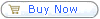5 Seat \$2,999Premium Support SubscriptionSingle \$299 More Information5 Seat \$999 Licensing Information Total SQL Statistics is licensed on a per developer basis. Each developer who uses or redistributes the program must have a license. Runtime/Redistributable Version Total SQL Statistics includes royalty-free redistribution rights.